• 实验三 MATLAB符号运算 一 实验目的 1.掌握符号对象的创建及符号表达式化简的基本方法 2.掌握符号微积分符号方程的求解的基本方法 二 实验装置 计算机 三 实验内容 1.符号对象的创建 (1) 建立符号变量 使用sym函数...
• 七、MATLAB符号计算 7.1 符号对象 符号对象的计算结果是一个更加精确的表达式。数值计算的结果往往是值。 7.1.1 符号对象的建立 符号对象名=sym(A)，A可以是数值常量、矩阵或数值表达式**（不加单引号），符号对象可...
• MATLAB中，提供了强大的符号运算功能，并且有...在MATLAB中，符号计算的函数主要分为以下几类:符号表达式、符号矩阵操作、符号微积分、符号积分变换、符号方程求解、符号函数的绘图、图形化符号函数计算器 符号...
在MATLAB中，提供了强大的符号运算功能，并且有专门的符号数学工具箱Symbolic Math Toolbox。此外，在MATLAB中，还可以通过maple. m和map. m两个接口和Maple相连。MATLAB的符号计算功能非常强大。在MATLAB中，符号计算的函数主要分为以下几类:符号表达式、符号矩阵操作、符号微积分、符号积分变换、符号方程求解、符号函数的绘图、图形化符号函数计算器
符号数学工具箱中定义了一种新的数据类型: sym类。sym类的实例就是符号对象，用来存储代表符号的字符串在MATLAB中，提供了两个建立符号对象的函数:函数sym( )和函数syms( ),两个函数的用法不同。
在MATLAB中，可以利用函数sym( )建立单个的符号变量。采用函数syms( )一次定义多个符号变量。此外，可以通过函数class()获取符号变量的类型。对于'r'，默认设置，为最接近的有理数表示；对于‘d’，为最接近的十进制浮点数32位表示；syms x y也可以定义两个符号变量。
在老版本的matlab可以直接用符号变量表达式，新版本会警告（我这里是matlab2016为例测试的）；
0.符号函数和符号方程
在MATLAB中，符号表达式是由符号常量、符号变量、符号函数运算符以及专用函数连接起来的符号对象。符号表达式有两类:符号函数和符号方程。方程与函数的区别为:函数是一个代数式, 而方程是一个等式。对于一元二次方程，, 其中a≠0,根据一元二次方程的求根公式,得到方程的两个根。当时，该方程有实根，该方程有实根;当时， 方程有两个共轭的复根。在MATLAB中,利用函数diff()来求导数。根据复合函数的求导公式进行计算。在MATLAB中，利用函数int( )求不定积分。在MATLAB中，利用函数int( )求不定积分。求解微分方程dy/dt=ay,该方程为可分离变量微分方程，进行变量分离，然后进行积分。采用函数dsolve( )求解微分方程。
>> x1 = solve('a*x^2+b*x+c=0');//方程求解

>> syms x;
>> y = diff(x^5*cos(x)+cos(pi/6));//表达式求导

>> syms x;
>> y = int(exp(sin(x))*cos(x),'x');//表达式进行不定积分

>> syms x;
>> y = int('sin(x)',x,0,pi);//表达式进行定积分
1.运算精度
在MATLAB中，采用函数digits( )和vpa( )来实现任意精度的符号运算。下面分别进行介绍:digits(d):调用该函数后，符号对象的近似解的精度变成d位有效数字,参数d的默认值为32位。D=digits:调用该函数后，得到当前采用的数值计算的精度。R=vpa(A):该函数计算符号矩阵A的近似解，精度为函数digits(d)指定的有效位数。R=vpa(A,d):该函数计算符号矩阵A的近似解，有效位数由参数d决定。
在MATLAB中，采用了函数重载技术，使得符号表达式的运算符和基本函数与数值计算中的运算符和基本函数几乎完全相同。符号表达式可以进行“+”、“-”、“*”、“/”四则运算。符号表达式的比较中，只有运算符“= =”和“~ ="代表“等于”和“不等于”。当结果为“真”时，返回值为1,否则返回值为0。
符号表达式的常用操作，包括:利用函数findsym( )寻找符号变量。利用函数factor()进行符号多项式的因式分解。利用函数expand()进行符号表达式的展开。利用函数collect( )进行符号表达式中同类项的合并。利用函数horner()将符号多项式转换成嵌套形式。利用函数numden( )获取符号表达式的分子和分母。
在MATLAB中，采用函数simplify( )和函数simple( )进行符号表达式的化简，下面分别进行介绍。函数simplify( )是一个具有普遍意义的工具,能够对包含和式、方根、分数的乘方、指数函数、对数函数、三角函数等的表达式进行化简。函数simple()也能进行符号表达式的化简，该方法比函数simplify()要简单，所得到的结果也比较合理。
在MATLAB中，可以采用函数subexpr( )和函数subs( )进行符号替换，让符号表达式的输出变的简单。函数subexpr()将符号表达式中重复出现的字符串用变量代替。函数subs()将符号表达式中重复出现的字符串用数值或字符串替换。
在MATLAB中，采用函数finverse( )进行反函数运算。该函数的调用格式为:g=finverse(f):该函数将会计算输入参数f的反函数,其中f为符号表达式，以默认的变量为自变量。函数的返回值g也是一个符号表达式。g=finverse(f, v):输入参数v是-一个符号变量，是函数f中的变量，且该函数的返回值g以v为自变量。
在数学计算中，经常会遇到求复合函数的情况。例如,函数z=f(y),而该函数的自变量y又是一个关于x的函数,即y=g(X)。此时，z=f(g(X)), z是关于x的一一个复合函数。在MATLAB中，采用函数compose( )进行符合函数运算。
2.符号矩阵
符号矩阵也是一种符号表达式，前面介绍的符号表达式运算都可以用于符号矩阵，需要注意的是这些函数作用于符号矩阵时，是分别作用于矩阵的每一个元素。由于符号矩阵是一个矩阵，所以符号矩阵还能进行有关矩阵的运算。此外，曾介绍过的许多应用于数值矩阵的函数，也可直接应用于符号矩阵。
在MATLAB中，可以采用函数sym( )直接产生符号矩阵,也可以将数值矩阵转换为符号矩阵。下面介绍如何生成符号矩阵：
1.采用函数sym()直接生成符号矩阵
2.利用函数sym()将数值型变量转换为符号型变量
对于符号变量和的四则运算有:A+B和A-B:实现符号矩阵的加法和减法。如果A和B为同类型的矩阵(具有相同的行数和列数)时，分别对对应的元素进行加减运算。如果A和B中有一个为标量，则矩阵中的每一个元素和该标量进行加法或减法。A*B:实现矩阵A和B的乘法，要求矩阵A的列数必须等于矩阵B的行数。如果A和B中有一个为标量，则将矩阵中的每一个元素乘以该标量。A\B:实现矩阵的左除法。X=AIB为符号线性方程组A*X=B的解。另外，A\B近似等于inv(A)*B。如果X不存在或不唯一，则系统显示警告信息。B/A:实现矩阵的右除法。X=B/A为符号线性方程组X*A=B的解。B/A近似为B*inv(A)。如果X不存在或不唯一，则系统显示警告信息。
符号矩阵和数值矩阵非常的类似，也可以求符号矩阵的转置秩、逆矩阵、行列式和特征值分解等。下面进行详细的介绍。
1.符号矩阵的转置2.符号方阵的幂运算3.符号矩阵的秩4.符号方阵的逆矩阵和行列式计算5.符号方阵的特征值分解6.约当标准型7.符号矩阵的奇异值分解
3.复杂运算
在MATLAB中，采用函数diff( )进行微分和求导运算,利用Jacobian函数jacobian( )实现对多元符号函数的求导。在MATLAB中，采用函数limit( )求符号表达式的极限。
在MATLAB中，采用函数int( )来计算符号表达式的不定积分和定积分。该函数的调用格式为:R=int(S):该函数计算S的不定积分,输入参数S可以是符号表达式或符号矩阵。没有指定积分变量和积分阶数，系统按函数findsym()得到的默认变量对S求不定积分。R=int(S, v):该函数对S中指定的符号变量v求不定积分。需要注意的是，函数的返回值R只是其中的一个原函数，后面没有带任意常数C。R=int(S, a, b):该函数计算S在闭区间[a, b]上的定积分。由于没有指定积分变量，系统按函数finldsym( )得到的默认变量计算定积分。a和b分别表示定积分的下限和上限,可以是两个具体的数，也可以是一一个符号表达式，还可以是无穷大(inf )。R=int(S, V, a, b):该函数计算S对于变量v在区间[a, b]上的定积分。
符号运算不仅能够求解方程,还可以求解方程组。下面介绍如何利用函数solve( )求解符号代数方程组，函数dsolve()求解微分方程组。在MATL AB中，利用函数solve( )求解一般符号代数方程组。该函数的调用格式为:solve('eqn1'):该函数求解单个的方程eqn1。solve('eqn1', 'eqn2', ... 'eqnN):该函数用来求解由N个方程组成的方程组。solve('eqn1', 'eqn2', ... 'eqnN', 'var1', 'var2', ... 'varN'):该函数中var1等表示符号变量，以这些变量为自变量,进行方程的求解。
在MATLAB中，采用函数dsolve()进行微分方程的求解。该函数的调用格式为:dsolve('eq1'):该函数对单个的微分方程eq1求解。dsolve('eq1, eq2, ..'‘cond1, cond2, ..’, ‘V'):该函数对由eq1,eq2等组成的微分方程组求解，初始条件为cond1, cond2deng，自变量为V。如果不指定参数v,则系统默认以t为自变量。
（0）级数求和
在MATLAB中，采用函数symsum( )进行级数符号的求和。该函数的调用格式为:symsum(S):该函数没有指定求和的符号变量，参数S表示级数的通项。symsum(S, V):该函数对变量v进行级数求和。symsum(S, a, b):该函数对默认变量从a到b进行级数求和。symsum(S, V, a, b):该函数对于变量v从a到b进行级数求和。
在MATLAB中，采用函数taylor( )求符号表达式的泰勒级数展开式，该函数的调用格式为:taylor(f):计算函数f在默认变量等于0处作默认为5阶的泰勒展开式。taylor(f,n):计算函数f在默认变量为0处做n-1阶的泰勒展开式的默认值为6。taylor(f,a):计算函数f在默认变量等于a处做默认为5阶的泰勒展开式，a的默认值为0。taylor(f, n, a):计算函数f在默认变量等于a处做n-1阶的泰勒展开式。taylor(f, n, v, a);计算函数f在变量等于a处做n-1阶的泰勒展开式，变量变为V。
（1）傅里叶变换
时域中的信号f(t)与它在频域中的Fourier变换F(w)之间存在如下的关系。

在MATLAB中，采用函数fourier( )计算傅立叶变换，采用函数ifourier( )计算傅立叶变换的反变换。
（2）拉普拉斯变换
对于函数f(x)进行拉普拉斯(Laplace)变换的公式为:

对函数L(s)进行拉普拉斯反变换的公式为:

在MATLAB中，实现拉普拉斯变换的函数为laplace(),进行拉普拉斯反变换的函数为ilaplace()。
（3）Z变换
对于离散序列f(n)的Z变换为：

对于F(z)的Z反变换为:

在MATLAB中，利用函数ztrans( )进行Z变换，利用函数iztrans( )进行Z反变换。
4.绘图
在MATLAB中，对于符号方程,可以非常方便的绘制其图形。本节将介绍符号函数的图形绘制，常用的绘图函数如表所示：
函数名函数的功能fplot一元函数绘图ezplot二元函数绘图ezpolar极坐标系下的绘图ezplot3三维图形ezmesh三维网格图ezmeshc带等值线的三维网格图ezcontour等值线图ezcontourf带填充的等值线图ezsurf三维彩色曲面图ezsurfc带等值线的三维彩色曲面图
在MATLAB中，提供了图形化的符号函数计算器。图形化的符号函数计算器功能虽然不是十分的强大，但是操作非常简单和方便，用户可以可以对符号运算和函数图形有个直观的了解。在MATLAB中，有两种符号函数计算器，单变量符号函数计算器和泰勒汲数逼近计算器，下面分别进行介绍。
在MATLAB中，可以使用函数funtool来调用图形化的单变量符号函数计算器。用户在命令行窗口中输入:funtool,可将单变量符号函数计算器调出，包括3个图形窗口。
在MATLAB中，可以使用函数taylortool来调用图形化的泰勒级数逼近计算器。用户在命令行窗口中输入:taylortool,即可将泰勒级数逼近计算器调出。泰勒级数逼近计算器是一个交互的界面，可以绘制函数f(x)前N阶的泰勒级数。系统默认的函数为f(x)=x*cos(x),泰勒级数展开的阶数为7，自变量的默认区间为[-2*pi, 2* pi]。
5.Maple接口
为了在MATLAB中进一步利用Maple的符号计算能力,MATLAB的符号数学工具箱提供了两个函数:函数sym()和函数maple( )。这两个函数能够实现对Maple中绝大多数符号计算函数的调用，利用它们可以扩充MATLAB的符号计算能力，下面分别进行介绍。
在MATLAB中，可以采用函数sym( )来调用Maple函数,来扩充MATLAB的符号计算功能。然后，通过函数subs( )进行变量的替换。
在MATLAB中，可以采用函数maple( )调用Maple中的函数。在MATLAB中可以通过函数mhelp来获得Maple函数的帮助信息。例如，在命令行输入: mhelp gcd,将获取Maple中的函数gcd的帮助信息。函数gcd()用于求两个多项式或整数的最大公约数。


展开全文• PAGE PAGE 1 实验三MATLAB符号计算 1已知x=6 , y=5利用符号表达式求; 2分解因式 (1) x4-y4 (2) 125x6+75x4+15x2+1 3化简表达式 (1)sin(1)cos(2)-cos(1)sin(2) ; (2) (4x2+8x+3)/(2x+1) 4已知 完成下列运算 (1) B=...
• 利用matlab求解下列各题 计算下列极限 1 2 3 2求下列函数的导数 1求的一阶导数 2设求 3已知求y' 3求下列积分 1 2 4解微分方程 5求的6阶Taylor展开式 6求方程在1附近的近似根 7设 求1, (2, (3)A的特征值
• 对于勒让德符号 (m/p)，p 必须是奇素数。 雅可比符号 (m/n) 允许 n 是任何奇数。
• 一、符号计算 1、符号计算特点  1、计算精确：符号计算基于数学公式、定理并通过一系列推理、演绎得到方程的解或者数学表达式的值。对操作对象不进行离散化和近似化处理。  2、可应用范围有限：实际科研和生产...
一、符号计算
1、符号计算特点
1、计算精确：符号计算基于数学公式、定理并通过一系列推理、演绎得到方程的解或者数学表达式的值。对操作对象不进行离散化和近似化处理。
2、可应用范围有限：实际科研和生产中遇到的问题绝大多数都无法获得精确的符号解，这时我们不得不求助数值计算。
3、对待符号计算态度：用其来完成公式推导和解决简单的对计算时效性要求不高的问题，综合符号计算和数值计算各自的优点，视问题特点混合使用符号计算和数值计算。

2、符号对象和符号表达式
1、符号对象的创建：要生成一个符号对象，可以利用sym以及syms函数，sym可以生成单个符号对象，而syms可以生成多个符号对象，符号对象的运算是完全精确的，没有舍入误差。例如：a = sym(‘5’);  syms b c d;。

2、符号表达式：创建了符号对象，我们就可以创建各种各样的符号表达式。譬如，创建符号变量a, b, c后如下都是符号表达式：
z1 = a+b+c;
z2 = sin(a+b+c);
z3= a^b*gamma(c);
确定一个符号表达式中的符号变量可以用findsym函数：
findsym(expr);
findsym(expr, n);
第一种用法是确认表达式expr中所有自由符号变量，第二种用法是从表达式expr中确认出距离x最近的n个符号变量。这个最近距离指的是变量第一个字符和x的ASCII码值之差的绝对值，差绝对值相同时，ASCII码值大的字符优先。findsym是老版本中的方法，新版本将使用symvar函数代替：symvar(expr);。

符号对象的比较中，没有“大于”、“大于等于”，“小于”，“小于等于”的概念，而只有是否“等于”的概念，即“==”与“~=”。如果要判断两个符号数值的大小一般来说有两种办法，一种是利用double将其转化成数值型的，另一种是利用sort+“==”或“~=”。譬如下面代码：

>> a = sym('2');  >> b= sym('3');  >>double(a)<double(b)  ans =  1  >> sa = sort([b,a])  sa =  [ 2, 3]  >>a==sa(1)  ans =  1

从上述代码可以看出，上述两种方法都间接实现了判断大小。

4、符号计算与数值计算结合：利用符号计算得到结果时，有时需要将其转化成数值型的以便后续数值计算利用。通过符号计算得到一个表达式时，想把它转化成关于其中某个变量的数值函数。
很多时候我们需要求符号表达式在不同的参数值下的具体值，说通俗点就是如何把具体的参数代入符号表达式。这时候可以利用eval和subs函数或者转化成匿名函数。
程序实例：

clc;  clear;   % 将符号变量转化成数值  format long;  % 可供使用的一些方法  % 这里a是符号变量，a1--a4是数值变量  a = vpa(pi,30); %3.14159265358979323846264338328  a1 = double(a); %3.141592653589793  a2 = eval(a); %3.141592653589793  a3 = single(a); %3.1415927  a4 = int8(a); % 3    % 很多时候我们需要求符号表达式在不同的参数值下的具体值，  % 说通俗点就是如何把具体的参数代入符号表达式。这时候可以利用eval和subs函数或者转化成匿名函数   % 如下的例子：求函数：f =sin( x^x / (x^2/exp(x)) ); 其二阶导数在x = 1处的值。  syms x;  f = sin(x^x / x^2/exp(x));  % 利用符号计算求f(x)的二阶导数  % diff函数用于求导数或者向量和矩阵的比较。  % 如果输入一个长度为n的一维向量，则该函数将会返回长度为n-1的向量，向量的值是原向量相邻元素的差  d2f = diff(f, x, 2);   % 第一种方法：利用subs函数求d2f在x=1时的值。  d2fx1 = subs(d2f, x, 1); % d2fx1 = 2.2082   % 第二种方法：x赋值1后，利用eval函数求d2f在x = 1时的值  x = 1;  d2_fx1 = eval(d2f); %d2_fx1 = 2.2082   % 第三种方法：将d2f转化成匿名函数，求其在x = 1时的值  % vectorize的含义就是将乘转成点乘等。 '*' -> '.*'； '/' -> './'； '^' -> '.^'； 最后再将替换结果中的“..”删除一个"."。  F = eval(['@(x)',vectorize(char(d2f))]);  F(1) % ans= 2.2082

二、极限、导数和级数的符号计算
1、求的极限。

clc;  clear;   % 求极限  syms n;  % limit函数用于求极限运算。假如y=f(x)，limit(y)表示x→0时的极限，limit(y,x,a)表示x→a时的极限  % MATLAB中gamma函数即数学上的gamma函数，当n为正整数时，有如下性质：gamma(n+1) = n!  limit( n^(n+1/2) /( exp(n)*gamma(n+1)), n,inf) % ans= 1/(2*pi)^(1/2)

2、求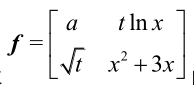的导数，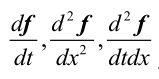。

% 求导数  syms a t x;  f = [a, t*log(x); sqrt(t), x^2+3*x];  % 矩阵f对t的一阶导数  dfdt = diff(f,t); % dfdt = [ 0, log(x); 1/(2*t^(1/2)), 0 ];   % 矩阵f对x的二阶导数，由于是x，而f中含有x变量，故x可以省略  dfdx2 = diff(f,2); % dfdx2 =[ 0, -t/x^2; 0, 2 ];   %求二阶混合导数  dfdtdx = diff(diff(f,t),x); %dfdtdx =[ 0, 1/x; 0, 0 ];

3、求无穷级数：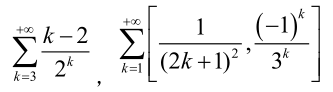% 求级数
syms k;
f1 = symsum( (k-2)/2^k, k, 3, inf);  % f1 = 1/2

A = [1/(2*k+1)^2, (-1)^k/3^k];
f2 = symsum(A, k, 1, inf); % f2= [ pi^2/8 - 1, -1/4]

三、求解方程
求解方程通常有两种方法，符号求解和数值求解。
1、solve
通常在不确定方程是否有符号解的时候，推荐先使用solve进行尝试，因为solve相比于数值求解来说，它不需要提供初值，并且一般情况下能够得到方程的所有解。对于一些简单的超越方程，solve还能够自动调用数值计算系统给出一个数值解。
solve的调用形式：
sol = solve(eq)
sol = solve(eq, var)
sol = solve(eq1, eq2, …, eqn)
sol = solve(eq1, eq2, …, eqn, var1, var2, …, varn)
eq为符号表达式，var为指定的要求解的变量。如果不声明要求解的变量(第一和第三种形式)，则matlab自动按默认变量进行求解，默认变量可以由symvar(eq)确定。
例：求解方程组: x+y=1, x-11y=5

% 使用solve函数求解方程  % 例：求解方程组: x+y=1, x-11y=5  clc;  clear;   % 声明符号变量  syms x y;  % 定义方程  eq1 = x + y -1;  eq2 = x - 11*y - 5;  % 调用solve求解方程组  % （solve函数的参数包括方程表达式，以及要求解的变量，这里变量是可选参数，不指定时matlab自动按默认变量进行求解）  % 这时候solve求得的解通过结构体的形式赋值给sol，然后再通过x=sol.x和y=sol.y分别赋值给x和y。  sol = solve(eq1, eq2, x, y);  x = sol.x;  y = sol.y;  % 也可以直接使用：  % [x,y] = solve(eq1, eq2, x, y);   % 使用double将符号解转换为数值解  value_x = double(x);  value_y = double(y);

需要注意，等式左边接收参数时应当按字母表进行排序，否则MATLAB不会自动识别你的参数顺序，比如：
[x, y] = solve(eq1, eq2, x, y)
[y, x] = solve(eq1, eq2, x, y)
solve会把答案按字母表进行排序后进行赋值，x解赋值给第一个参数，y解赋值给第二个参数，那么对于第二种形式，实际上最终结果是变量y存储了x的解而变量x存储了y的解。
由于是符号求解，有时候得到的解是一大串式子(符号求解无精度损失，所以MATLAB不会自动将答案转化为浮点数)，这时候可以用vpa或者double函数将结果转换为单一的数。
另外很多人习惯对于solve的参数采用字符型输入，这种方式有几个弊端，首先就是程序的调试，一旦式子输入有误(最常见的就是括号的配平)，将会对程序调试带来很大的困难。
其次是采用字符型输入时，对变量的赋值并不能传入方程，以x+y*sin(x)=1这个方程为例：y = 1;  sol = solve('x+y*sin(x)=1','x')，MATLAB会返回一个空解，但是对于sym型输入：syms x;  y=1;  eq=x+y*sin(x)-1;  sol=solve(eq,x); 能够得到sol=0.5109734293885691，其中的区别就在于char型输入尽管在solve前对y有一个赋值，但solve求解时依然会将y当作一个未赋值的常数。
最后，在高版本solve已经不支持char型参数输入，因此应该放弃使用这种方法。

2、fzero
然而在很多情况下solve并不能求得方程的解析解，这时就可以采用数值法求解。
数值求解法包括fzero和fsolve，其区别在于fzero只适用求解一元函数零点，而fsolve适用于求解多元函数零点(包括一元函数)。当求解一元函数零点时，推荐优先使用fzero，原因是fzero求解一元方程往往更容易，因为它不仅支持提供初值的搜索，还支持在一个区间上进行搜索。
fzero的常用形式：
x = fzero(fun, x0);
[x, fval] = fzero(fun, x0);
其中fun为函数句柄，x0为搜索初值，fval为求解误差。
如果方程有多个零点时，fzero只能根据提供的初值求得最靠近初值的一个零点，如果希望求得多个零点的话，那么只能够通过改变初值来得到不同的零点。
对于零点的选取，目前来说没有什么比较好的办法，只能够通过分析方程的性质，或者通过作图的方法去寻找一个比较靠近零点的初值。另外，fzero能够提供区间搜索，注意区间两端的端点函数值符号需要反向。
以一元方程sin(x)+cos(x)^2=0为例：

clc;  clear;   % fzero只适用求解一元函数零点  % 求sin(x)+cos(x)^2=0的零点  % 这里采用匿名函数，也可以使用函数文件形式  y = @(x)sin(x)+cos(x).^2;  % 1为搜索初值，fval为求解误差  [x, fval] = fzero(y, 1);   % fzero在[3, 4]这个区间搜索初值  [t, eval] = fzero(y,[3 4]);

3、fsolve
fsolve可以求解多元方程，用法和fzero类似。
fsolve的常用形式：
x = fsolve(fun, x0);
[x, fval] = fsolve(fun, x0);
其中fun为函数句柄，x0为搜索初值，fval为求解误差。
例：求解方程组x+y=1, x-11y=5

% fsolve函数求解多元函数  % x+y=1, x-11y=5  eq = @(x)[x(1)+x(2)-1; x(1)-11*x(2)-5];  [sol, err] = fsolve(eq, [1,1]);

这里对于方程的的输入需要采用矩阵的形式，其中x(1)代表x，x(2)代表y。有时候变量较多时可能会容易混淆，这里提供另一种方法，采用符号变量形式再利用matlabFunction转化为函数句柄：

% 采用符号变量形式再利用matlabFunction转化为函数句柄  syms x y  eq1 = x+y-1;  eq2 = x-11*y-5;  % 将符号函数转化为函数句柄  eq1 = matlabFunction(eq1);  eq2 = matlabFunction(eq2);  eq = @(x)[eq1(x(1), x(2)); eq2(x(1), x(2))];  [sol2, err2] = fsolve(eq, [1,1]);

效果与之前相同，但不容易出错。求得的解以矩阵形式返回给sol，即sol的第一个值是匿名函数的第一个输入参数值x，sol的第二个值是匿名函数的第二个输入参数值y。

4、vpasolve
vpasolve是R2012b引进的函数，可以求解一元或多元函数零点。相比于fzero和fsolve来说，vpasolve最大的一个优点就是不需要提供初值，且能够自动搜索指定范围内的多个解。
vpasolve调用形式：
S = vpasolve(eqn);
S = vpasolve(eqn, var);
S = vpasolve(eqn, var, init_guess);
___ = vpasolve(___, Name, Value);
其中eqn是符号方程，var为需要求解的变量，也可以不提供(第一种形式，这是默认求解变量由symvar(eqn)求得)，init_guess为搜索初值，Name, Value为一些选项控制。
例：对于多项式方程，vpasolve能够给出所有解：

syms x;  vpasolve(4*x^4 + 3*x^3 + 2*x^2 + x + 5 == 0, x);  ans =  - 0.88011 - 0.76332i  0.50511 + 0.81599i  0.50511 - 0.81599i  - 0.88011 + 0.76332i

对于非多项式方程，vpasolve给出它找到的第一个解：

syms x;  vpasolve(sin(x^2) == 1/2, x);  ans =  -226.94

这时可以提供搜索初值，来改变它找到的解：

syms x;  vpasolve(sin(x^2) == 1/2, x,100);  ans =  99.996

可以指定搜索范围，但不同于solve，solve指定求解范围是用assume函数，vpasolve则是直接在输入参数中指定：

syms x;
vpasolve(x^8 - x^2 == 3, x, [-Inf Inf]); %实数范围内求解

最后，vpasolve一个很强大的用法，将‘random’选项设置为true可以直接搜索指定范围内不同解：

syms x;  f = x-tan(x);  for n = 1:3  vpasolve(f,x,'random',true);  end

5、左除”\”与右除”/”
在MATLAB环境中，强烈建议使用左除”\”或者右除”/”解线性方程组。左除和右除是根据除号左侧还是右侧是分母而定的，方程系数矩阵在未知数左侧，则用左除，反之用右除。使用左除”\”或者右除”/”的好处是因为其对线性方程(组)的广泛适用性，当未知数个数大于方程个数的时候，左除或右除会给出方程的特解，结合null函数，可以得到通解。当未知数个数小于方程个数的时候，左除或右除会给出方程的最小二乘解。

四、求解积分
求解积分与求解方程相同，也有两种方法，符号求解和数值求解。与数值积分相比，符号积分具有指令简单，占用机时长等特点，因此一般复杂的积分运算都采用数值积分函数来计算。但某些情况下，特别是一些简单的上下限为函数的多重积分，用符号积分计算会比调用数值积分函数计算简单方便许多。

1、int
int是符号积分求解器，调用形式简单，但是功能非常强大。
int常用形式：
intf = int(expr, var)   %不指定积分上下限，即求解不定积分
intf = int(expr, var, a, b)    %指定积分上下限，即求解定积分
上述调用格式中var可以省略，var省略时，积分将针对findsym确定的变量来进行。a, b作为积分上下限，实际输入中可以为数值符号或者字母符号。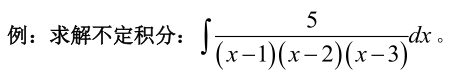% int求积分  syms x;  % 这里没有制定区间，求解的是不定积分  f = 5 / ((x-1)*(x-2)*(x-3));  F = int(f, x); % F = (5*log(x^2 - 4*x + 3))/2 -5*log(x - 2)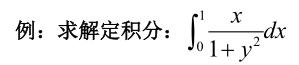syms y;  f = x / (1 + y^2);  F = int(f, y, 0, 1); % F = (pi*x)/4

但是大多情况下int都得不到解析解，这时候就可以采用数值积分。

2、integral
q = integral(fun, xmin, xmax);
q = integral(fun, xmin, xmax, Name, Value);
其中fun为函数句柄，xmin为积分下限，xmax为积分上限，Name和Value是一些选项控制，包括误差、向量化积分等等。integral配合fzero可以求解无法显式表达的函数的定积分。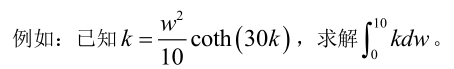% integral求数值积分  q = @(k, w)w.^2 / 10.*coth(30*k)-k;  v = @(w)fzero(@(k)q(k,w), 1e3); % 利用fzero求解k, 相当于显式表达k  value = integral(v, 0, 10, 'ArrayValued', 1);

3、trapz
trapz是基于梯形法则的离散点积分函数。调用形式：
I = trapz(x, y);
其中x和y分别是自变量和对应函数值。
例：sin(x)在[0, pi]的积分：

% trapz求离散点积分  t = linspace(0, pi, 1e3); %生成[0, pi]内的一系列离散点  y = sin(t);  I = trapz(t, y);


展开全文• 09 : 圆周率的计算章。 10 - 11：Lin.Alg。 （MATLAB），应用程序。 章。 12：格斯特。 数据 (MuPAD) 章。 13：方程（MuPAD） 章。 14 : Lin.Alg。 （MATLAB，MuPAD） 章。 15 : 微积分 (MuPAD) 章。 16 - 19 : ...
• 此脚本将计算“对称”Pade' 的系数，该系数近似于在 x=0 处展开的符号函数。 它还返回极点和零点以确保扩展在感兴趣的区域中没有不良行为。 用户可以自由修改和重新分发此脚本。
• MATLAB编程符号计算 行命令a=sym('pi','d')，则对于变量a的描述 分别使用 sym 和syms创建符号表达式“sin(x)+cos(y)”
• 使用matlab进行符号定积分运算如下： syms x; syms b; f =b + 2*x; a=int(f,x,-6,6) a =12*b 首先设置参量，本例中为x 和 b； int函数（被积函数，积分变量，积分下限，积分上限），如果只有一个参量，则可以...
使用matlab进行符号定积分运算如下：
   syms x;
syms b;
f =b + 2*x;
a=int(f,x,-6,6)

a =12*b
首先设置参量，本例中为x 和 b； int函数（被积函数，积分变量，积分下限，积分上限），如果只有一个参量，则可以省略第二项。上下两例分别对x和b进行积分。
   syms x;
syms b;
f =b + 2*x;
a=int(f,b,-6,6)

a =24*x
展开全文• MATLAB课件2007-第七讲 MATLAB符号计算.ppt matlab全面综合讲解课件
• Matlab符号计算，需要用到一些常用符号工具箱函数，例如画出 f(x)=x2f(x)=x^2f(x)=x2 在 [0,3][0,3][0,3] 上的微分值： \qquad(1) 首先构造符号变量x，符号表达式f(x)=x^2，再利用工具箱函数 df=diff(f) 直接求出...
Matlab的符号计算，需要用到一些常用符号工具箱函数

例如画出

f

(

x

)

=

x

2

f(x)=x^2

在

[

0

,

3

]

[0,3]

上的微分值：

(1) 首先构造符号变量x，符号表达式f(x) = x^2；再利用工具箱函数 df = diff(f) 直接求出其导数为 df = 2*x。因此，符号计算的过程不会产生误差。

(2) 微分值可以直接使用工具箱函数subs(df,x1)或者subs(df,x,x1)，其中x代表符号表达式df中所采用的符号变量（或者通过symvar(df)自动获取），x1 为待求解的点

多变量函数在涉及到需要采用不同符号变量的时候，可以采用subs(df,symvar(df),x1)的方式

(3) 通过vpa(x,n) 设置符号数x的精度为n

(4) 最后将符号数强制类型转换为浮点型数值

其中，(2)~(4) 综合在一起就是val = double(vpa(subs(df,symvar(df),x0),4));
% symbolic
syms x
f2 = x^2
figure(2),ezplot(f2,[0,3]);hold on;
x1 = 0:0.1:3;
val2 = diff1_s(f2, x1);
plot(x1,val2)
legend('x^2','2x')

function val = diff1_s(f, x1)
df = diff(f);   % symbolic math toolbox
val = double(vpa(subs(df,symvar(df),x1),4));
end

运行结果：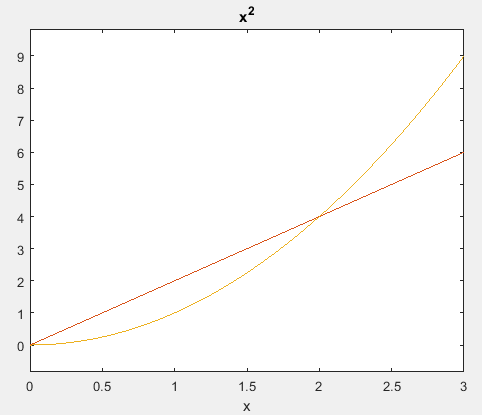Matlab的数值计算，可以采用“匿名函数”作为函数的参数。

相应的数学运算（例如积分、微分），需要编写对应的数值算法，例如画出

f

(

x

)

=

x

2

f(x)=x^2

在

[

0

,

3

]

[0,3]

上的微分值：

(1) 微分运算可以采用“中心差分”的公式，因此数值计算包含了计算误差

(2) 由于是数组运算，定义匿名函数时采用了“点乘”的方式，配合数值运算的需要
% numerical
x1 = 0:0.1:3;
f1 = @(x)(x.^2)
figure(1)
plot(x1,f1(x1));hold on;
val1 = diff1_c(f1,x1,0.1);
plot(x1,val1);
legend('x^2','2x')

function val = diff1_c(f,x,h)
val = (f(x+h)-f(x-h))/(2*h);
end

运行结果：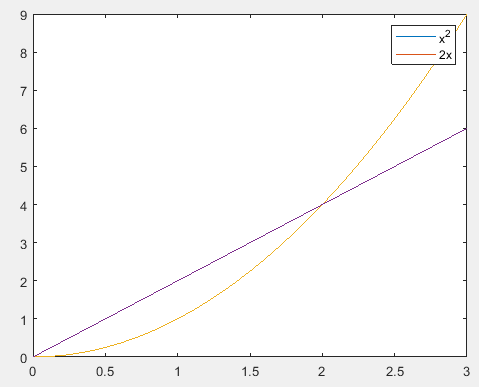展开全文• 计算消息中的符号概率将有助于在实际发送编码之前先进行编码。
• 什么是微分方程？如下； 如下图，也是一个微分方程； matlab使用 dsolve 命令解微分方程； 下面来解 dy/dx=4*x^3 这个方程； 一阶，二阶，多阶，都可以解；微分方程 微积分 dsolve
• ## MATLAB求符号函数的函数值的方法

万次阅读 多人点赞 2018-05-05 14:49:29
MATLAB中定义函数的方法有许多种 ，比较常用的一种是定义符号变量 x 和 y。举一个简单的例子：对函数 y = x^2 ,用上述方法的MATLAB语言如下：syms x y y=x^2; 要想画出函数图像使用 ezplot() 函数，其中[-20,20]...
• 文件是MATLAB学习过程中自己总结的符号计算部分思维导图，方便复习使用，有png和mmax版，方便用思维导图软件编辑
• 本人收集的MATLAB基础教程-第9章 MATLAB符号计算.ppt 第13章 在Word环境下使用MATLAB.ppt 第12章 Simulink动态仿真集成环境.ppt 第11章 MATLAB图形用户...
• 练习 7 求不定积分 练习 8 用数值与符号两种方法求给定函数的定积 分并对结果进行比较 7.5 级数符号求和 ? 级数求和 ? 有穷级数求和 sum ? 借助于符号表达式求和函数 symsum 可以 对有穷级数无穷级数求和 ? 调用格式...
• MATLAB编程与仿真 MATLAB与科学计算 第2章 MATLAB符号计算.ppt MATLAB编程与仿真 MATLAB与科学计算 第3章_数组及向量化运算.ppt MATLAB编程与仿真 MATLAB与科学计算 第4章 数值计算.ppt MATLAB编程与仿真 MATLAB与...
• MATLAB符号计算(MATLAB程序设计教程电子教案) 9.1 符号对象 9.1.1 建立符号对象 1建立符号变量和符号常量 MATLAB提供了两个建立符号对象的函数sym和syms两个函数的用法不同 (1) sym函数 sym函数用来建立单个符号量...
• 理解符号变量、符号表达式、符号矩阵等概念，掌握符号矩阵和符号表达式的创建，了解符号运算与数值运算的不同点，会修改已有的符号矩阵，并会符号矩阵与数值矩阵的相互转换，掌握符号矩阵矩阵的运算。熟练掌握符号求...
• 这是一个利用MATLAB符号函数功能，通过手动输入DH参数符号矩阵 or Excel文件导入DH参数...通过该代码获得的DH矩阵不仅能够再次利用matlab计算所需变化矩阵，甚至还能将具体数值带入求得的转换矩阵中求得DH矩阵具体值。
• MATLAB 符号工具箱参考书籍，数学微积分计算MATLAB 符号工具箱参考书籍，数学微积分计算
• 符号变量及其运算绪：什么是符号计算？1.字符型数据变量的创建2.符号型数据变量的创建3.符号计算的运算符与函数4.寻找符号变量5.符号精度计算6.显示符号表达式7.合并符号表达式8.展开符号表达式 绪：什么是符号计算...矩阵
• MATLAB程序设计教程 MATLAB与高等数学 第09章 MATLAB符号计算（共23页）.ppt MATLAB程序设计教程 MATLAB与高等数学 第10章 MATLAB图形句柄（共13页）.ppt MATLAB程序设计教程 MATLAB与高等数学 第11章 MATLAB图形......

# matlab计算符号matlab 订阅